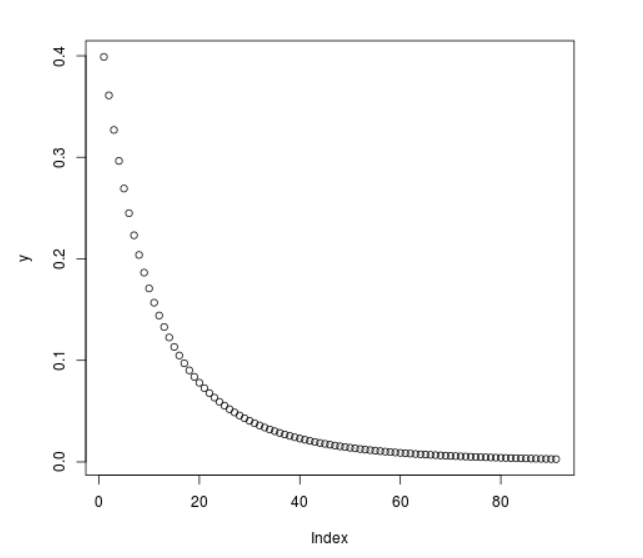Open in App
Not now

# Compute Log Normal Probability Density in R Programming – dlnorm() Function

• Last Updated : 25 Jun, 2020

`dlnorm()` function in R Language is used to compute the log normal value of the probability density function. It also creates a plot of the log normal density.

Syntax: dlnorm(vec)

Parameters:
vec: x-values for normal density

Example 1:

 `# R program to compute``# log normal probability density`` ` `# Creating x-values for density``x <``-` `seq(``1``, ``10``, by ``=` `1``)`` ` `# Calling dlnorm() function``y <``-` `dlnorm(x)``y`

Output:

```  0.398942280 0.156874019 0.072728256 0.038153457 0.021850715 0.013354538
 0.008581626 0.005739296 0.003965747 0.002815902
```

Example 2:

 `# R program to compute``# log normal probability density`` ` `# Creating x-values for density``x <``-` `seq(``1``, ``10``, by ``=` `0.1``)`` ` `# Calling dlnorm() function``y <``-` `dlnorm(x)`` ` `# Plot a graph``plot(y)`

Output:My Personal Notes arrow_drop_up